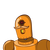# In the given figure , O is the centre of the circle and ACB is inscribed in arc ACB. If ACB = 650

In the given figure ,
O is the centre of the circle and
ACB is inscribed in arc ACB.
If ACB = 650

, then find m(arcAPB).

### 1 thought on “In the given figure ,<br /> O is the centre of the circle and<br /> ACB is inscribed in arc ACB.<br /> If ACB = 650<br /><br />”

1.## 一═デ︻ αηѕωєя ︻デ═一

m(arc ACB)=230°

Explanation:

Given : ∠ACB is inscribed in a arc ACB of a circle with centre O.

∠ACB =65°

The Inscribed Angle Theorem : The measure of an inscribed angle is half the measure the arc intercepted by it.

Therefore , ∠ACB= half of Measure of arc AB

⇒Measure of arc AB = 2 x  ∠ACB = 2 x 65° =130°

Since arcAB is minor arc and arc ACb is major arc and tyhe sum of minor and major arc is 360°.

⇒ Measure of Major arc = 360°- Minor arc

⇒ Measure of arc ACB = 360°  -130°

=230°

Hence, m(arc ACB) =230°